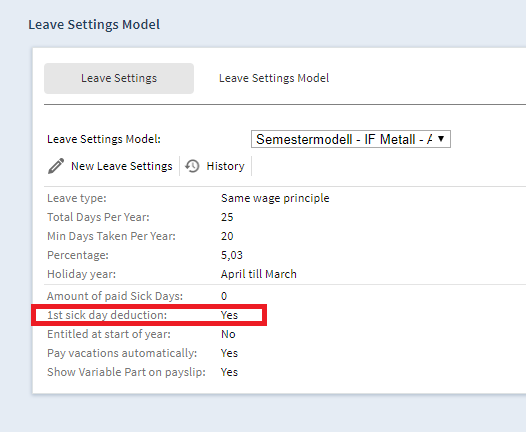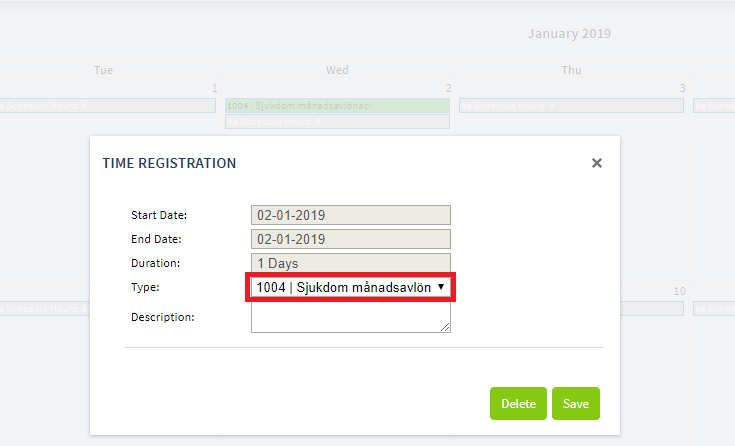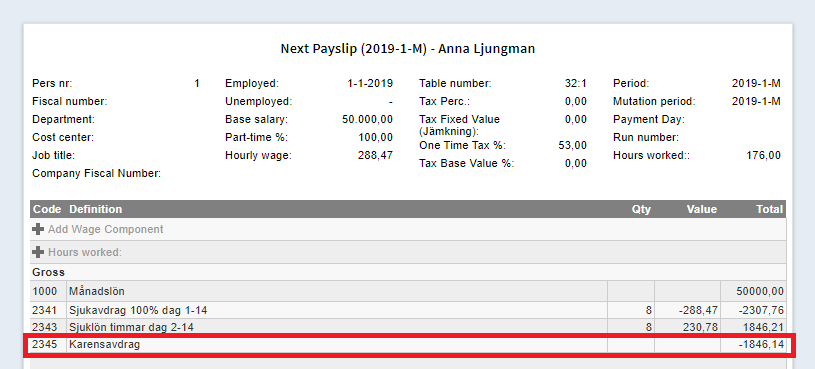# First day of sickness (Karensavdrag)

Swedish version of the article

In the leave model there is a setting called 1st sick day deduction (Karensavdrag). When this setting is true in years before 2019, the first day of sickness is unpaid.

From 2019 onwards there is a new calculation for this. If an employee has a 1st sick day deduction then he gets a deduction on his gross payment, called karensavdrag. This will be deducted on wage code 2345.

### Setting in leave modelThe 1st sick day deduction in the leave model that belongs to the company has to be true.

### Sickness day in the time registrationAdd a sickness day in the time registration on code 1004 (the whole day) or code 1005 (hours).

### Calculation on the payslipThe 1st sick day deduction will be calculated based on the percentage of sick pay, days per week, base 10305 in the reports and periods per year.

1. The percentage of sick pay = 0,8.
2. 20% = factor 0,2 (if an employee works 40 hours a week and his first day of sickness is 8 hours, then 8 is 20% out of 40.)
3. Base 10305 (check reports) = 50.000.
4. Periods per year / 52 = 12 /52

The following calculation takes place:
0,8 * 0,2 * 50,000 * 12 /52 = 1846,15.

But next to that also a maximum amount will be calculated. The following variables are used for this calculation.

1. Input hours = hours of sick period in current period that overlap with employee schedule = 8
2. Percentage of sick pay = 0,8
3. Average schedule hours = employee schedule hours per week * 52 / periods per year = 173,33
4. Base 10305 = 50.000

The following calculation takes place:
8 * 0,8 * (50.000 / 173,33333, rounded on 2 decimals) = 1846,14.

Because the maximum is lower than the amount that is calculated, the max. will be applied. See payslip.